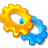# libssrckdtree Generic k-d tree C++ template librarylibssrckdtree is a C++ header-only template library of spatial data structures, currently containing only an implementation of a k-d tree largely conforming to STL associative container requirements. Additional spatial data structures may be added in the future. An older version of the software also included a Java implementation, which, by popular demand, has been repackaged as a separate libssrckdtree-j release.

libssrckdtree implements a fully generalized multi-dimensional k-d tree. You can use keys with two, three, or more dimensions and can store any value that can be stored in an STL container. Nearest neighbor and k-nearest neighbor search are implemented as of version 1.0.6.

# A Simple Example

The `kd_tree` template class follows the interface of STL map as far as possible, making it usable with some STL algorithms. However, it implements custom functions for range searching and optimizing end of range detection during iteration. Keys must be compatible with the TR1 fixed size array class, supporting both dynamic value retrieval via `operator[]` and compile-time value retrieval via `std::get`. It is not possible to use a tuple as a key, given dynamic value retrieval requirements during tree traversal. Container elements are stored by value, per STL requirements. Any class that can be stored in an STL container can be stored as a `kd_tree` mapped type.

The following example inserts some point/string pairs into a `kd_tree` instance, demonstrates the use of an STL algorithm, and iterates over a range to print some key-value pairs.

#include <ssrc/spatial/kd_tree.h> #include <ssrc/wispers/utility/ToString.h> #include <cstdlib> #include <ctime> #include <iostream> #include <algorithm> #include <cassert> template<typename T> struct TruePred { bool operator()(const T &) { return true; } }; typedef std::array<unsigned int, 2> Point; typedef ssrc::spatial::kd_tree<Point, std::string> Tree; typedef TruePred<Tree::value_type> True; inline std::ostream & operator<<(std::ostream & out, const Point & point) { return out << "[ " << point << ", " << point << " ]"; } int main(int argc, char *argv[]) { Tree tree; ssrc::wispers::utility::ToString to_string; std::srand(std::time(0)); for(unsigned int i = 0; i < 100; ++i) { Point point{ { std::rand() % 100, std::rand() % 100 } }; tree[point] = to_string(point); } assert((tree.size() == std::count_if<Tree::iterator, True>(tree.begin(), tree.end(), True()))); Point lower{ { 25, 25 } }, upper{ { 75, 75 } }; for(Tree::const_iterator it = tree.begin(lower, upper), end = tree.end(); it != end; ++it) { std::cout << it->first << " : " << it->second << std::endl; } /* Alternatively, the above loop can be written using the non-standard and * more efficient kd_tree::iterator::end_of_range() function. For example: * for(const_iterator it = tree.begin(lower, upper); !it.end_of_range(); ++it) */ return 0; }

# Unsupported Sava Algorithms Release

Sava Algorithms was something of an experiment and is not actively maintained. We make it available for those who require a Java implementation or need to use a C++ compiler that does not support C++0x. The Java implementation has been superseded by libssrckdtree-j.

 Version Java Source Java Binaries C++ Source License 0.1.1 sava-0.1.1-src.tar.gz sava-0.1.1-bin.tar.gz libsava-0.1.1.tar.gz LICENSE

 Java API Java Unit Test API C++ API Java Test Code Coverage Report C++ Test Code Coverage Report